Function Repository Resource:

# PooledStandardDeviation

Compute the pooled standard deviation of multiple lists of data

Contributed by: Seth J. Chandler
 ResourceFunction["PooledStandardDeviation"][{l1,l2,…}] computes a weighted norm of the standard deviations of each list li in which the weights are proportionate to the lengths of the constituent lists minus one.

## Details and Options

ResourceFunction["PooledStandardDeviation"] will not evaluate unless each list li has length greater than 1.

## Examples

### Basic Examples (2)

Compute the pooled standard deviation of two lists of data:

 In:=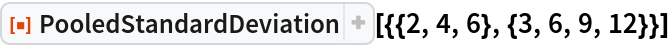Out=Compute the pooled standard deviation of symbolic data, assuming that the elements of the data are real:

 In:=Out=### Scope (2)

PooledStandardDeviation can work on collections of any finite length:

 In:=Out=PooledStandardDeviation can work on complex values:

 In:=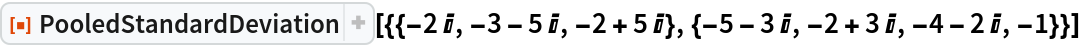Out=### Applications (1)

Compute Hedge’s g statistic, which makes use of the pooled standard deviation to measure the effect size for the difference between means:

 In:=Out=### Properties and Relations (2)

The pooled variance, which can be computed using the resource function PooledVariance, can also be computed by taking the square of the pooled standard deviation. Indeed, this is precisely how PooledStandardDeviation performs its computation:

 In:=Out=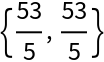The pooled standard deviation of a collection is not the same as the standard deviation of the flattened collection, even if the lengths of all the elements of the collection are the same:

 In:=Out=### Possible Issues (1)

PooledStandardDeviation will not evaluate unless each inner list has a length greater than 1:

 In:=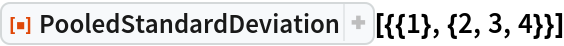Out=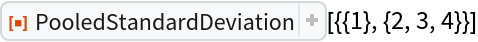Seth J. Chandler

## Version History

• 1.0.0 – 27 December 2019